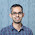## Wednesday, 11 July 2012

### Ping Pong game

Suppose A and B are equally strong ping pong players. Is the probability of A beating B in 3 out of 4 games is same as that of he beating in 6 out of 8 games?

#### 4 comments:

1.first one is 4C3/2^4 and second is 8C6/2^8

2.now what came in my mind is what will be the probability of a player winning half a time if they played infinitely...and intuitively that should be 1 as both are equally strong... so i calculated the limiting value of 2nCn/2^2n for n -> infinity ... 2nCn/2^2n = (1-1/2n) * (1-1/2(n-1)) * ... * (1 - 1/2*2) * (1-1/2*1) ... so as n -> infinity, the 1st term goes to 1, but all the terms are strictly less than 1 and so the limit value < 1 ..... (also 2nC < 2^2n for all n) .... so intuitively the probability should be 1 but limit value < 1 ... can anyone please help me what's wrong?

1.A gaussian doesn't necessarily have a peak value of 1 if it is normalized. It's not a delta function, there's a spread. And the number of possible outcomes goes to infinity.

2.Taking the infinite limit is going from a mass function to a density function, right? So I guess the probability of each individual result will go to zero, like it does in this case. We can only talk about ranges of outcomes